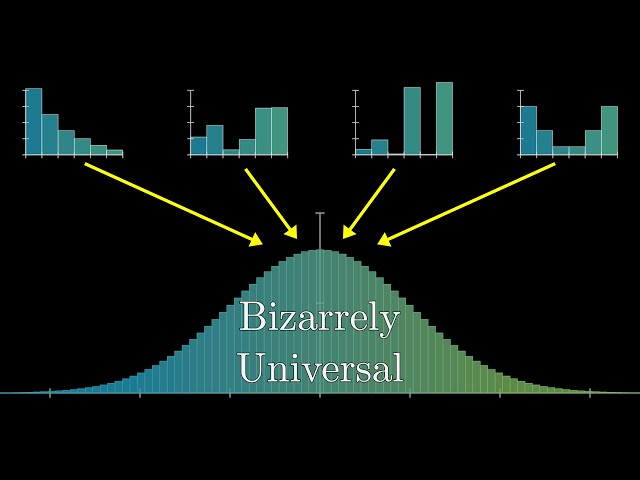# MathematicsMathematics Python

#### Python vs Wolfram Alpha, which one wins?

This video is from Everyday Mathematics. We move to the regular season of the MIT integration BEE and solve problems that bear log functions in them. We compare python and Wolfram alpha and can you guess which ones comes top?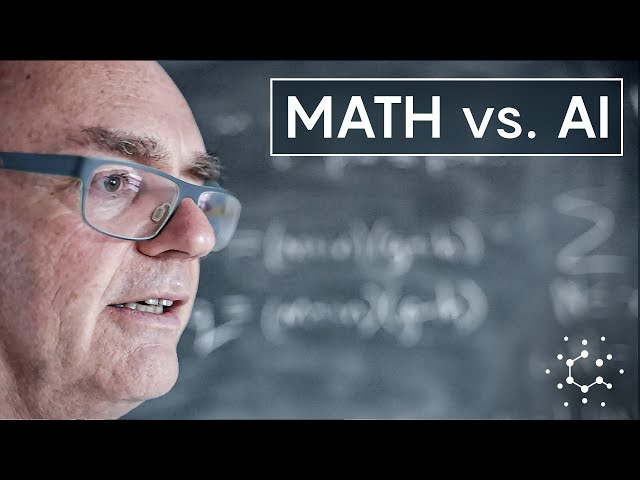AI Mathematics

#### When Computers Write Proofs, What’s the Point of Mathematicians?

Andrew Granville knows that artificial intelligence will profoundly change math. The programming language Lean already plays a role in theorem proving. That’s why the University of Montreal number theorist has started talking to philosophers about the nature of mathematical proof — and how the discipline of mathematics might evolve in the age of AI. Read […]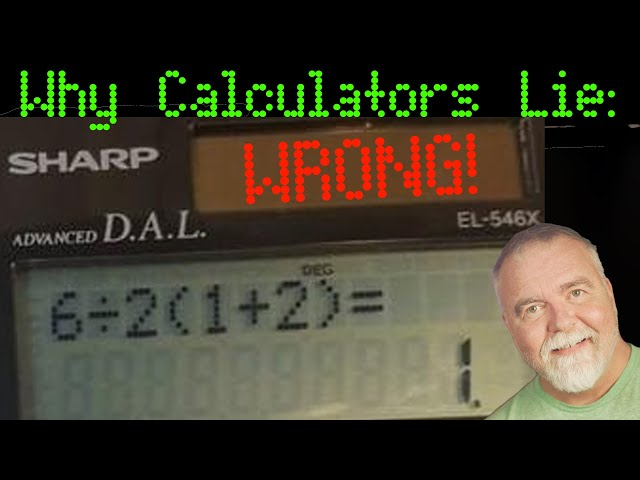Interesting Mathematics

#### Why Calculators Lie: Can You Solve This Simple Math Problem?

Dave takes us on a journey beginning with an incredibly simple math problem that many people – and even calculators – get wrong.AI Data Mathematics Privacy Security

#### How to Safely Share Secrets: Homomorphic Encryption in Open Source Explained

Ever wondered how your data can be computed without being exposed? Sounds impossible, right? Dive into the world of Homomorphic Encryption, the groundbreaking technique that promises data privacy like never before! In this video, we’ll decode the complexities of this encryption method and explore its significance in the open-source realm. Whether you’re a cryptography enthusiast, […]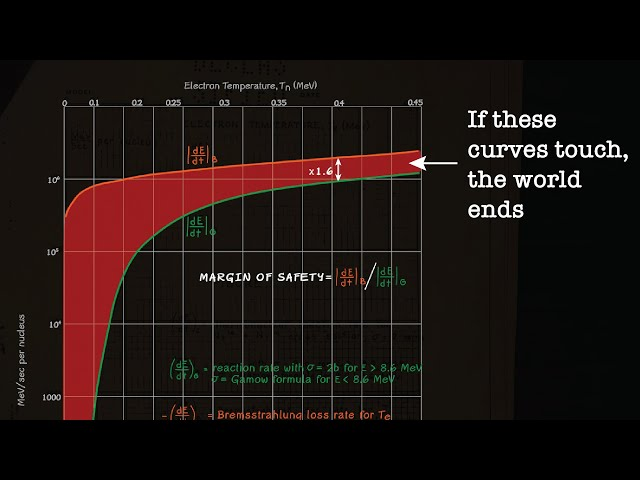Mathematics Science

#### Oppenheimer’s Apocalypse Math

Welch Labs highlights the Los Alamos Report 602, which conjectured that if a fusion bomb big enough could ignite the entire atmosphere.Interesting Mathematics

#### Did You Know About the 200-year-old mathematics behind half the internet

This video is from Improbable Matter discusses how Fourier Transforms – breaking up signals into individual waves – allows lossy compression of sound, images and movies like the one you are watching now!Mathematics

#### Discover a Revolutionary Way to Do Math

There’s a strange number system, featured in the work of a dozen Fields Medalists, that helps solve problems that are intractable with real numbers. Veritasium explains.Mathematics

#### Uncover the Hidden Connection Between Thousands of Unsolved Math Problems

Up and Atom finds the link between the unsolved mysteries of math.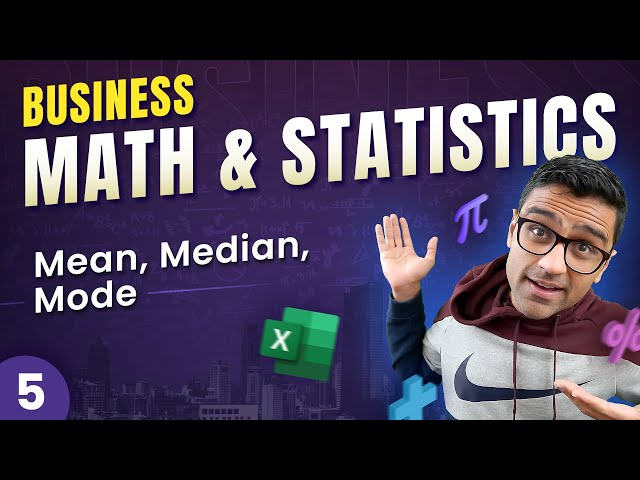Mathematics Statistics

#### How to Do Business Math & Statistics with Excel – Calculate Mean, Median & Mode

This video from codebasics explains how to do basic statistical analysis in Excel.. Mean, median and mode are basic statistical formulas that businesses use on daily basis to make data-informed decisions. In this video, I will give you a very simple explanation of these concepts using a few business use cases. We will also use […]